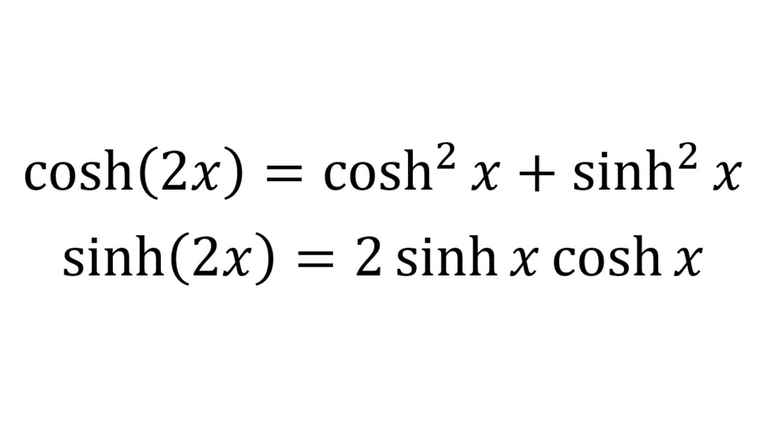# Hyperbolic Trigonometric Identity: cosh(2x) & sinh(2x)

in Threespeak2 months ago (edited)In this video I go over the derivations of the double angle (or double argument) identities for hyperbolic trig cosine and sine, namely cosh(2x) and sinh(2x). The derivation of both is pretty straight forward given that 2x = x + x, and thus we can simply replace the y in x + y for both sinh(x+y) and cosh(x+y) identities which I solved in my earlier videos. The resulting identities are:

cosh(2x) = cosh^2(x) + sinh^2(x)
sinh(2x) = 2sinh(x)cosh(x)

I will be utilizing these hyperbolic trigonometry identities so make sure to watch this video and understand how they are derived!

Download the notes in my video: https://1drv.ms/b/s!As32ynv0LoaIhv1nc17jrUe5xLwUiA

View video notes on the Hive blockchain: https://peakd.com/mathematics/@mes/video-notes-hyperbolic-trigonometric-identity-cosh-2x-and-sinh-2x

Related Videos:

Hyperbolic Trigonometric Identity: cosh(x+y): https://youtu.be/B_rfrnhx-t4
Hyperbolic Trigonometric Identity: sinh(x+y): https://youtu.be/CtBzwqd4Rqc
Hyperbolic Functions - tanh(x), sinh(x), cosh(x) - Introduction: http://youtu.be/EmJKuQBEdlc .

SUBSCRIBE via EMAIL: https://mes.fm/subscribe

DONATE! ʕ •ᴥ•ʔ https://mes.fm/donate

Like, Subscribe, Favorite, and Comment Below!

Follow us on:

MES Truth: https://mes.fm/truth
Official Website: https://MES.fm
Hive: https://peakd.com/@mes
Gab: https://gab.ai/matheasysolutions
Minds: https://minds.com/matheasysolutions
Twitter: https://twitter.com/MathEasySolns
Facebook: https://fb.com/MathEasySolutions
LinkedIn: https://mes.fm/linkedin
Pinterest: https://pinterest.com/MathEasySolns
Instagram: https://instagram.com/MathEasySolutions
Email me: [email protected]

Free Calculators: https://mes.fm/calculators

BMI Calculator: https://bmicalculator.mes.fm
Grade Calculator: https://gradecalculator.mes.fm
Mortgage Calculator: https://mortgagecalculator.mes.fm
Percentage Calculator: https://percentagecalculator.mes.fm

Free Online Tools: https://mes.fm/tools

iPhone and Android Apps: https://mes.fm/mobile-apps

▶️ 3Speak